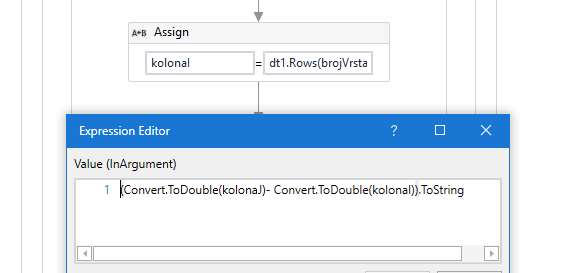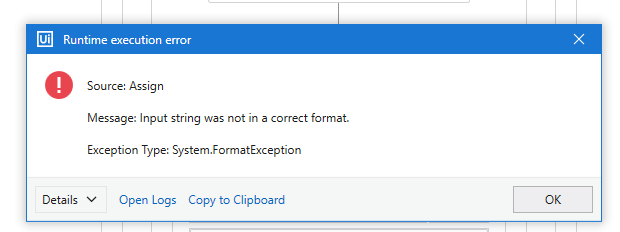# Subtract two column

hello

I have a table and I need to subtract two columns (J-I) and write the result in column (K). I’ve tried it like thisBut it tells me an error.Hi @dvojinovic ,

Could you check what are the values of the variables ?

@dvojinovic
can you show use some more part of code

Try this

2. Passed the DT1 in for each loop.
3. Inside the loop assign
Currentrow(“Result”)=Cdbl(currentrow(“Column1”).tostring)-Cdbl(Currentrow(“Column2”).toString)
4.Outside the loop write range Dt1.

I hope it helps!!

2. Passed the DT1 in for each loop.
3. Inside the loop assign
Assign double var1=row(“K”)
var1= Convert.ToDouble(row(“J”)) - Convert.ToDouble(row(“I”))
4.Outside the loop write range Dt1.

Hope it helps!!

The Error was pointing out that the data to convert to double is not in a numeric format.

So, Checking if the value extracted from the datatable contains numeric value or not could be a start to understand what needs to be done further.

You can check the values by Debugging or writing the value to the Output Panel using Write Line activity or even with a Message Box. Just make sure to check the value.

Debugging is a better option, it would also be helpful for you in further developments. A Tutorial is mentioned below :